Equivalent Fractions – Two Worksheets

Equivalent Fractions Worksheets

Fraction

Equivalent Fractions – Equivalent Fractions Example – Equivalent Fractions Meaning

Equivalent Fractions -Equivalent fractions are equal to each other. Two fractions are equal if they represent the same portion of a whole.

For example,

1/2=2/4=4/8

and

1/2=2/4=4/8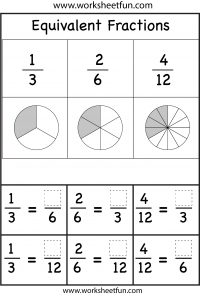1/3=2/6=4/12

Find the equivalent fractions.

More Equivalent Fractions Worksheets

### New Worksheets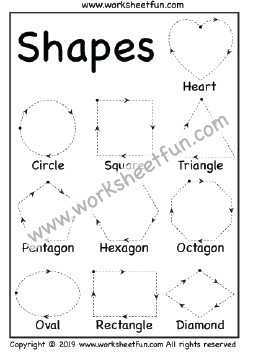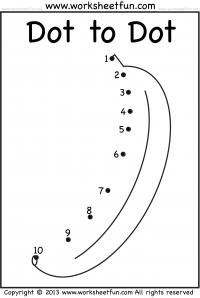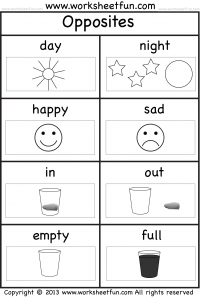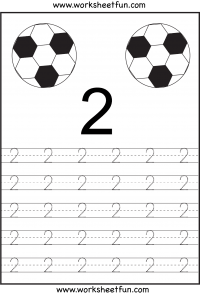### Most Popular Preschool and Kindergarten Worksheets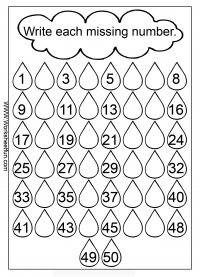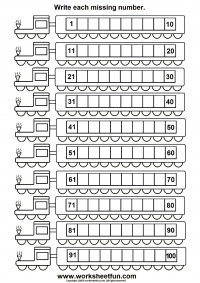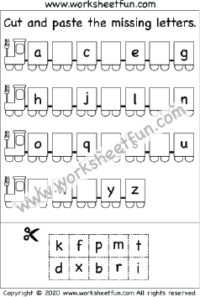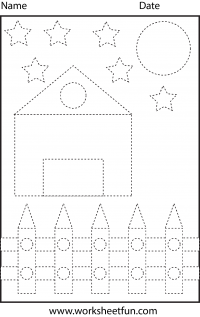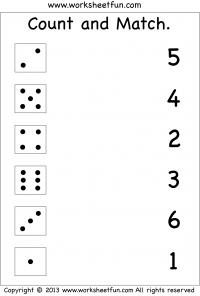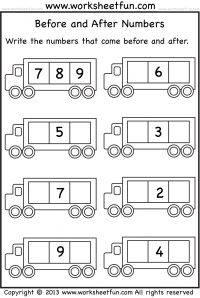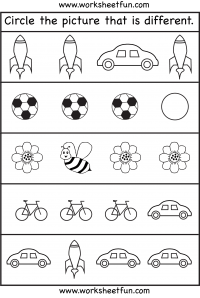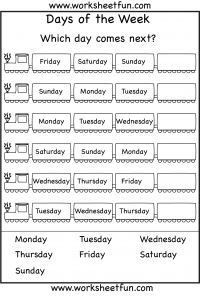#### Kindergarten Worksheets